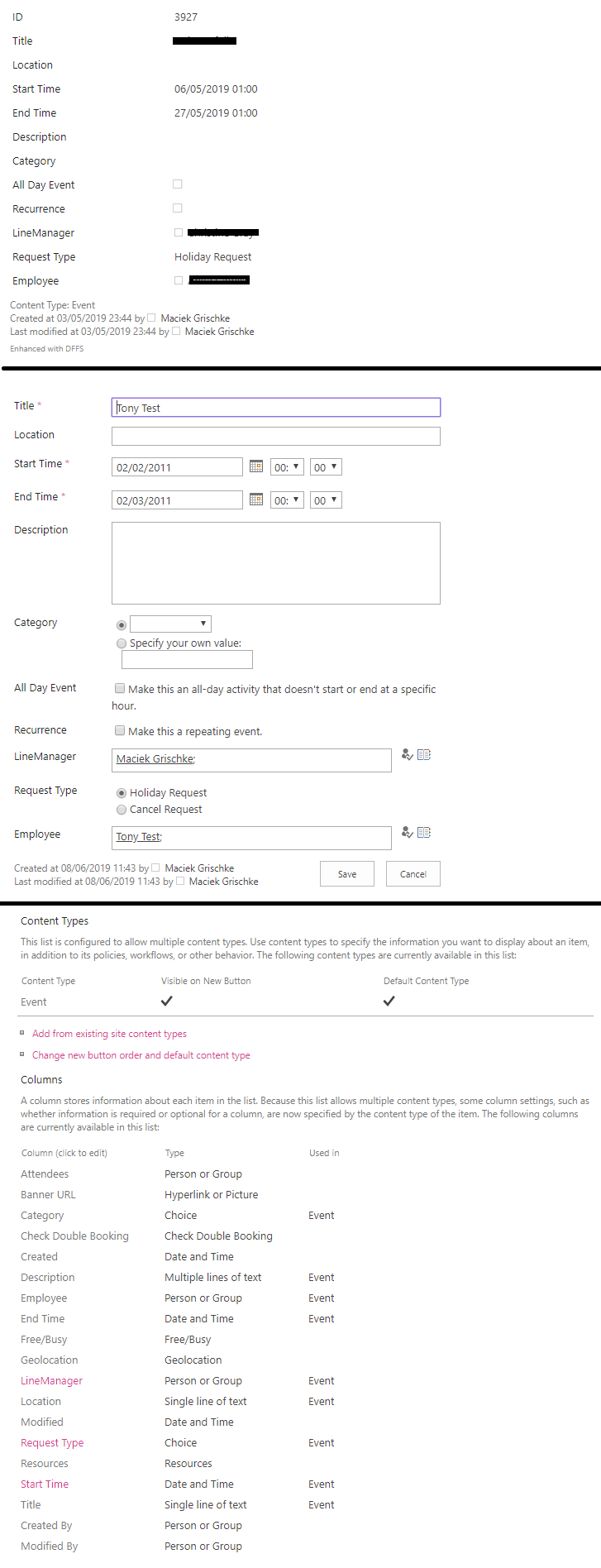# Calculate time difference in hours or minutes

Forums Classic DFFS Calculate time difference in hours or minutes

• Author
Posts
• #25577

How can I calculate the time difference between two Date and Time fields?

I’m struggling to convert the format “21/03/2012 12:00” to milliseconds in JS.

Perhaps DFFS has a built-in function that will simply calculate the time difference?

Basically, I have two date & time fields: Start Date and End Date.

For example, I want to be able to calculate:
Start Date & Time – End Date & Time
01/06/2019 7:30 – 01/06/2019 22:00 as 14.5 hours, or
01/06/2019 22:00 – 02/06/2019 7:30 as 9.5 hours

I can do it in calculated columns no problem, but I want to display it in the form in real time for users to see the calculation. I can do this in JS, but I have difficulties with JS date formatting.

I’d appreciate any help with this.

• #25579

Ok, I found a way to do this, but maybe there’s a better way?

```var startDate = "03/06/2019 20:15";
var endDate = "03/06/2019 22:00";
//console.log("startDate");
//console.log(startDate);
var startDate2 = startDate.split("/");
var endDate2 = endDate.split("/");
var startDate3 = startDate2 + "/" + startDate2 + "/" +startDate2;
var endDate3 = endDate2 + "/" + endDate2 + "/" +endDate2;
//console.log("startDate3");
//console.log(startDate3);
var startDate4 = new Date(startDate3);
var endDate4 = new Date(endDate3);
//console.log("startDate4");
//console.log(Date.parse(startDate4));
//console.log("endDate");
//console.log(Date.parse(endDate4));
var calc = (endDate4 - startDate4)/1000/60/60;
console.log(calc);
```

I just need help getting this to work in NewForm when Date and Time is selected 😉
I’d like to enter the total hours in “Total” field.

• #25585

Hi,

```function dateChanged(){
var d1 = spjs.utility.getDateFieldAsDateObject("DateColumn1");
var d2 = spjs.utility.getDateFieldAsDateObject("DateColumn2");
if(d1 !== "" && d2 !== ""){
var ms = d2-d1;
var hours = (ms / (1000 * 60 * 60)).toFixed(2);
setFieldValue("TargetField",hours);
}
}```

Then add a rule to each of your date fields triggering on change, and add the function name dateChanged in the Run these functions / trigger these rules section.

Let me know how this works out.

Alexander

• #25591

Thanks Alexander, this works great in standard lists, but not calendar.

I get “invalid date” and NaN as the result.

Dates in Calendar are set as Event columns. Not sure what the difference is, because the calculated column can calculate dates no problem.

• #25596

Can you send me (or attach) a screenshot of the calendar with the date field so I can see how it looks?

Please remove any sensitive info from the screenshot.

Alexander

• #25636

Here’s the screenshot(s):This is a “calendar” list.

• #25642

This is really strange – for some reason SharePoint uses a different format for the value of the hour in the calendar vs a custom list. If you inspect the hour in a custom list it shows a value from 0-23, but in a calendar it shows the value in the same way as the text 0-12 AM/PM. This causes the spjs-utility function to fail converting the string to a proper date object.

I’ll fix this in the next version of DFFS, but in the meantime you can add this function to you Custom JS (above where you use the function:

```spjs.utility.getDateFieldAsDateObject = function (fin) {
var dateVal, hour = 12, minute = 0, dateArr, lcid, format, fArr, sep, date, y, m, d;
dateVal = spjs.\$("#dffs_" + fin).find("input:text").val();
if (dateVal !== "") {
if (spjs.\$("#dffs_" + fin).find("select").length > 0) {
hour = parseInt(spjs.\$("#dffs_" + fin).find("select:first").val(),10);
minute = spjs.\$("#dffs_" + fin).find("select:last").val();
}
lcid = spjs.\$("#dffs_" + fin).find("a[onclick^='clickDatePicker']").attr("onclick").match(/lcid=([^&]*)/);
format = spjs.dffs.data.lcidToDateFormat[lcid];
sep = format.match(/[^dmy]+/).toString();
fArr = format.split(sep);
dateArr = dateVal.split(sep);
y = Number(dateArr[fArr.indexOf("y")]);
m = Number(dateArr[fArr.indexOf("m")]);
d = Number(dateArr[fArr.indexOf("d")]);
date = new Date(y, m - 1, d, hour, minute, 0);
return date;
} else {
return "";
}
};```

Alexander